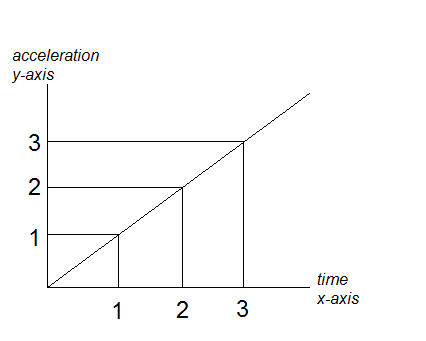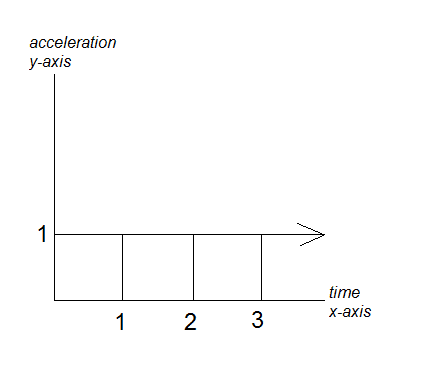# Building up to understand integrals/area under curve.

LearninDaMath

## Homework Statement

A particle starts at rest, then accelerates at a constant rate of 1 meter per second squared.

Perhaps a(t) = t

## The Attempt at a Solution

I have a series of calculus-related questions based on this statement, but first, I just want to know if the equation above, a(t)=t, and the graph below correctly represents the statement, "A particle starts at rest, then accelerates at a constant rate of 1 meter per second squared."EDIT:

Or, is the correct equation for the statement: a(t) = 1

and the graph for the statement:Last edited:

What you are asking is to solve the second-order differential equation $\displaystyle \frac{d^2 y}{dt^2}=1$. Luckily for you, solving this differential equation requires near to no work compared to other second-order equations.